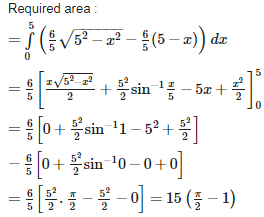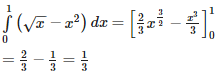# Application of Integrals - Online Test

Q1. The area of the region between the curve y = 4 –  and the x –axis is equal to
Explaination / Solution:

y =   And
Required area:
=  =

Q2. The area bounded by the curve  and the x –axis is
Explaination / Solution:

The given curve consists of two straight lines x + y = 1 ( x ≥ 0 )and -x + y = 1 ( x < 0 )
Required area :
= == = 1sq.unit

Q3. The area enclosed by the curve is equal to
Explaination / Solution:

The area of the standard ellipse is given by ;πab. Here , a = 5 and b = 4 Therefore , the area of curve is π(5)(4)=20π.

Q4. The area of the curve  is
Explaination / Solution:

Given equation is of a circle ;  Its radius is equal to a. Therefore required area is =
Q5. The area bounded by the curve y = 4x -  and the x- axis is equal to
Explaination / Solution:

For x – axis , y = 0,
Therefore,
Therefore , x = 0 or x = 4.
Required area :
=  = 32 - - 0 =
Q6. The area bounded by the curve and y – axis is equal to
Explaination / Solution:

Required area :

=sq.units

Q7. The area bounded by y = log x , the x – axis and the ordinates x = 1 and x = 2 is
Explaination / Solution:

Required area :
=  = = =

Q8. Area bounded by the curves satisfying the conditions  is given by
Explaination / Solution:Q9. The area bounded by the parabola y =  + 1 and the straight line x + y = 3 is given by
Explaination / Solution:

The two curves parabola and the line meet where,
3 - x =  = 0 $.\phantom{\rule{0ex}{0ex}}$
Required area ;
=
Q10. The area bounded by the curves  = x andy =  is
Explaination / Solution:

The two curves meet in ( 0 , 0 ) and ( 1, 1 ).The required area lies above the curve y = x2 and below x = y2 and is equal to ;